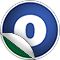# Conjunction

### Related Docs: class Conjunction | package conjunctions

#### object Conjunction

Linear Supertypes
AnyRef, Any
Ordering
1. Alphabetic
2. By inheritance
Inherited
1. Conjunction
2. AnyRef
3. Any
1. Hide All
2. Show all
Visibility
1. Public
2. All

### Value Members

1. #### final def !=(arg0: Any): Boolean

Definition Classes
AnyRef → Any
2. #### final def ##(): Int

Definition Classes
AnyRef → Any
3. #### final def ==(arg0: Any): Boolean

Definition Classes
AnyRef → Any

6. #### def apply(quans: Seq[Quantifier], arithConj: ArithConj, predConj: PredConj, negatedConjs: NegatedConjunctions, order: TermOrder): Conjunction

Create a `Conjunction` from an arbitrary collection of formulas.

Create a `Conjunction` from an arbitrary collection of formulas. It is required that all given formulas are sorted by the specified `order`.

9. #### def apply(quans: Seq[Quantifier], formulas: Iterator[Formula], logger: ComputationLogger, order: TermOrder): Conjunction

Create a `Conjunction` from an arbitrary collection of formulas.

Create a `Conjunction` from an arbitrary collection of formulas. It is required that all given formulas are sorted by the specified `order`.

10. #### final def asInstanceOf[T0]: T0

Definition Classes
Any
11. #### def clone(): AnyRef

Attributes
protected[java.lang]
Definition Classes
AnyRef
Annotations
@throws( ... )
12. #### def collectQuantifiers(f: Formula): Set[Quantifier]

Default: divisibility is not counted (but we count immediately preceeding quantifiers)

13. #### def collectQuantifiers(f: Formula, divCollector: (Conjunction) ⇒ Set[Quantifier]): Set[Quantifier]

Determine the quantifiers that occur in a formula.

Determine the quantifiers that occur in a formula. Because there are different points of view, a function can be given as parameter that determines whether (quantified) divisibility/indivisibility statements are counted as quantifiers

14. #### def compare(c1: Conjunction, c2: Conjunction, order: TermOrder): Int

Rudimentary sorting of conjunctions.

Rudimentary sorting of conjunctions. TODO: improve this (a lot)

17. #### def conj(formulas: Iterator[Formula], order: TermOrder): Conjunction

Compute a conjunction from an arbitrary set of formulas

18. #### def conjOrdering(order: TermOrder): Ordering[Conjunction]

Rudimentary sorting of conjunctions.

Rudimentary sorting of conjunctions. TODO: improve this (a lot)

19. #### def createFromNormalised(quans: Seq[Quantifier], arithConj: ArithConj, predConj: PredConj, negatedConjs: NegatedConjunctions, order: TermOrder): Conjunction

Create a `Conjunction` from different parts that are already normalised.

Create a `Conjunction` from different parts that are already normalised. This primarily means that `negatedConjs` must not contain any conjunctions that are just literals, and that quantifiers are pulled out completely if the conjunction only has a single conjunct.

23. #### def disjFor(formulas: Iterator[Formula], order: TermOrder): Conjunction

Compute a disjunction from an arbitrary set of formulas

24. #### final def eq(arg0: AnyRef): Boolean

Definition Classes
AnyRef
25. #### def equals(arg0: Any): Boolean

Definition Classes
AnyRef → Any
26. #### def eqv(for1: Formula, for2: Formula, order: TermOrder): Conjunction

Form the equivalence between two formulas

27. #### def finalize(): Unit

Attributes
protected[java.lang]
Definition Classes
AnyRef
Annotations
@throws( classOf[java.lang.Throwable] )
28. #### final def getClass(): Class[_]

Definition Classes
AnyRef → Any
29. #### def hashCode(): Int

Definition Classes
AnyRef → Any
30. #### def implies(for1: Formula, for2: Formula, order: TermOrder): Conjunction

Form the implication between two formulas

31. #### final def isInstanceOf[T0]: Boolean

Definition Classes
Any
32. #### final def ne(arg0: AnyRef): Boolean

Definition Classes
AnyRef
33. #### def negate(f: Formula, order: TermOrder): Conjunction

Negate a formula

34. #### final def notify(): Unit

Definition Classes
AnyRef
35. #### final def notifyAll(): Unit

Definition Classes
AnyRef
36. #### def quantify(quan: Quantifier, constants: Seq[ConstantTerm], f: Formula, order: TermOrder): Conjunction

Quantify a number of constants in a formula, i.e., replace the constants with variables and add quantifiers

37. #### def quantify(quans: Seq[Quantifier], f: Formula, order: TermOrder): Conjunction

Quantify a formula

38. #### def reverseConjOrdering(order: TermOrder): Ordering[Conjunction]

Rudimentary reverse sorting of conjunctions.

Rudimentary reverse sorting of conjunctions. TODO: improve this (a lot)

39. #### final def synchronized[T0](arg0: ⇒ T0): T0

Definition Classes
AnyRef
40. #### def toString(): String

Definition Classes
AnyRef → Any
41. #### final def wait(): Unit

Definition Classes
AnyRef
Annotations
@throws( ... )
42. #### final def wait(arg0: Long, arg1: Int): Unit

Definition Classes
AnyRef
Annotations
@throws( ... )
43. #### final def wait(arg0: Long): Unit

Definition Classes
AnyRef
Annotations
@throws( ... )